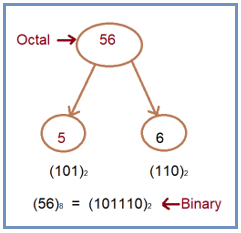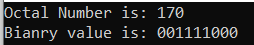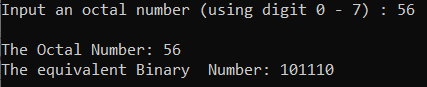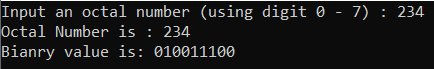# Octal to Binary Conversion in C#

## Octal to Binary Conversion in C# with Examples

In this article, I am going to discuss Octal to Binary Conversion in C# with Examples. Please read our previous article, where we discussed Hexadecimal to Octal Conversion in C#. At the end of this article, you will understand, what are Octal and Binary Number Systems are and how to convert an Octal Number into its equivalent Binary Number using C#.

##### What is Octal Number System?

The octal numeral system or oct is the base-8 number system and uses the digits 0 to 7. Octal numerals can be made from binary numerals by grouping consecutive binary digits into groups of three (starting from the right). Example: (5303)8

##### What is Binary Number System?

The binary numeral system uses the number 2 as its base (radix). As a base-2 numeral system, it consists of only two numbers 0 and 1. The binary number system is the way to represent the number in computer architecture. Example: (101101)2

##### Logic to Convert Octal to Binary in C#

To find the conversion of octal to decimal number simply we need to follow the below steps

1. First, we take the octal number.
2. Then we convert it as a decimal equivalent.
3. We convert the decimal number into their binary equivalent.
4. Then we store the number and we get the required binary number.
##### Example: Convert to octal number (56)8 into binary

Solution:
Given octal number is (56)8
The equivalent binary number of 5 is: 101
The equivalent binary number of 6 is: 110

Equivalent binary number of (56)8 is (101110)2

For better understanding, please have a look at the below diagram which shows how to convert (56)8 into a binary number.##### Example: Octal to Binary Number Conversion in C#

The following C# Program will convert an Octal number to its equivalent Binary number.

```using System;
class OctalToBinary
{
static void Main()
{
string octalNumber = "170";
System.Console.WriteLine("Octal Number is: " + octalNumber);
int i = 0;
string binary = "";
while (i < octalNumber.Length)
{
char c = octalNumber[i];
switch (c)
{
case '0':
binary += "000";
break;
case '1':
binary += "001";
break;
case '2':
binary += "010";
break;
case '3':
binary += "011";
break;
case '4':
binary += "100";
break;
case '5':
binary += "101";
break;
case '6':
binary += "110";
break;
case '7':
binary += "111";
break;
default:
System.Console.WriteLine("\nInvalid Octal Digit " + octalNumber[i]);
break;
}
i++;
}
System.Console.WriteLine("Bianry value is: " + binary);
}
}
```
###### Output:##### Example: Taking input from the user

In the following C# program, we will take the octal number from the user and then convert the octal number to its equivalent binary number and print it on the console.

```using System;
public class OctalToBinary
{
public static void Main()
{
long n1, n2, k = 1;
long decimal_number = 0, i = 1, j, d;
long binary_number = 0;
Console.Write("Input an octal number (using digit 0 - 7) : ");
n2 = n1;
for (j = n1; j > 0; j /= 10)
{
d = j % 10;
if (i == 1)
k *= 1;
else
k *= 8;

decimal_number = decimal_number + (d * k);
i++;
}
i = 1;

for (j = decimal_number; j > 0; j /= 2)
{
binary_number = binary_number + (decimal_number % 2) * i;
i *= 10;
decimal_number /= 2;
}

Console.Write("\nThe Octal Number: {0}\nThe equivalent Binary  Number: {1} \n\n", n2, binary_number);
}
}
```
###### Output:##### C# Code (Using function)

In the below example, we have created one method to convert the octal number to its equivalent binary number.

```using System;
class OctalToBinary
{
static string octalToBinary(string octalNumber)
{
int i = 0;
string binary = "";
while (i < octalNumber.Length)
{
char c = octalNumber[i];
switch (c)
{
case '0':
binary += "000";
break;
case '1':
binary += "001";
break;
case '2':
binary += "010";
break;
case '3':
binary += "011";
break;
case '4':
binary += "100";
break;
case '5':
binary += "101";
break;
case '6':
binary += "110";
break;
case '7':
binary += "111";
break;
default:
System.Console.WriteLine("\nInvalid Octal Digit " + octalNumber[i]);
break;
}
i++;
}

return binary;
}
static void Main()
{
Console.Write("Input an octal number (using digit 0 - 7) : ");